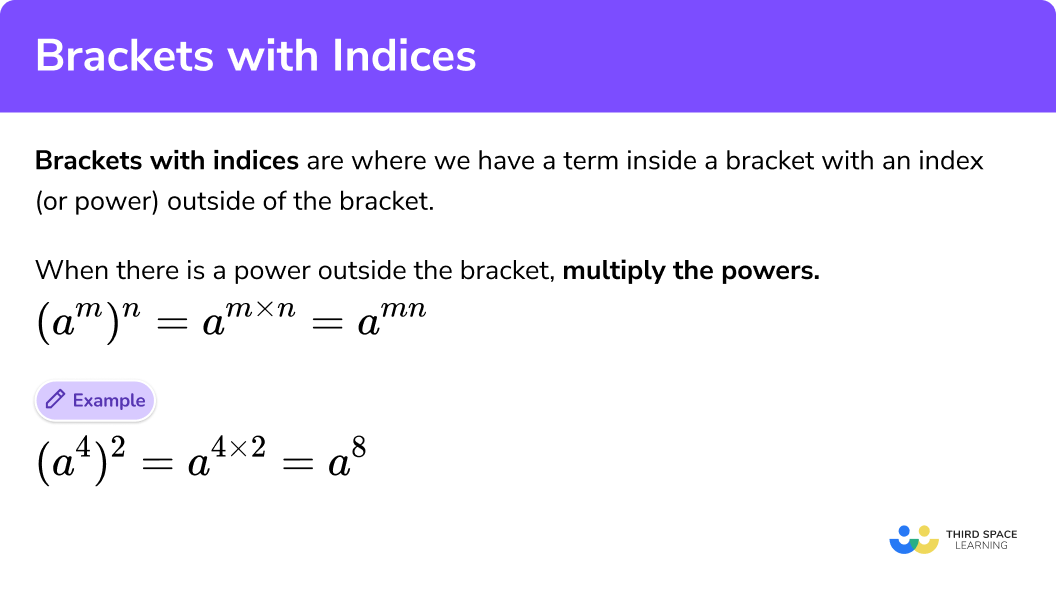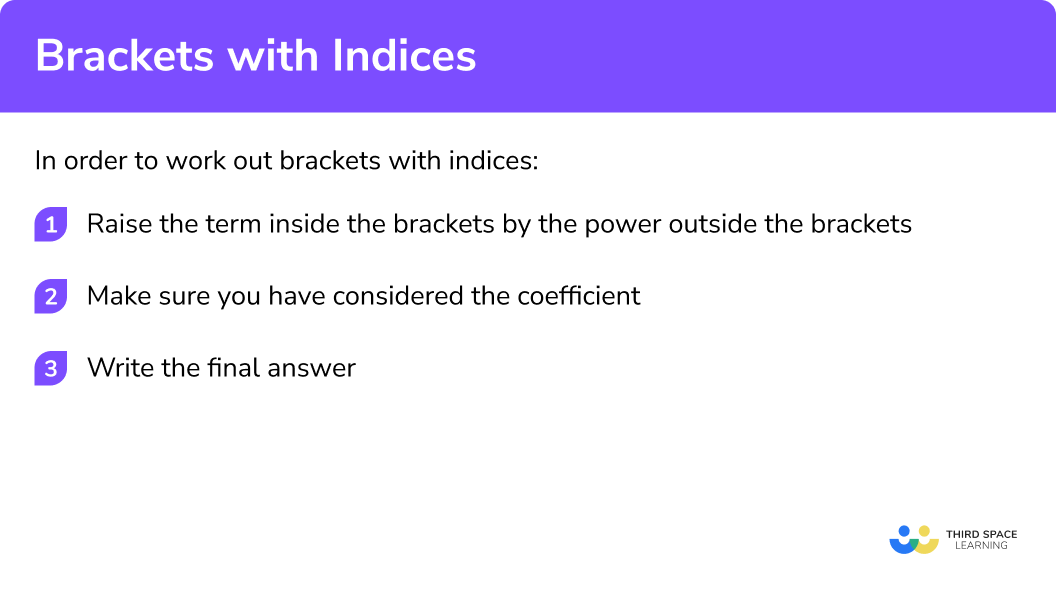GCSE Maths Algebra Laws Of Indices

Brackets With Indices

# Brackets With Indices

Here we will learn about brackets with indices.

There are also laws of indices worksheets based on Edexcel, AQA and OCR exam questions, along with further guidance on where to go next if you’re still stuck.

## What are brackets with indices?

Brackets with indices are where we have a term inside a bracket with an index (or power) outside of the bracket.
To do this we can raise everything inside the bracket to the power.

E.g.

$(a^{4})^{2}={a}^{4}\times{a}^{4}={a}\times{a}\times{a}\times{a}\times{a}\times{a}\times{a}\times{a}=a^{8}$

We could also have used the multiplication law of indices

$(a^{4})^{2}={a}^{4}\times{a}^{4}={a}^{4+4}=a^{8}$

However, a quicker method would be to multiply the indices:

$(a^{4})^{2}={a}^{4\times2}={a}^{8}$

In general when there is a term inside a bracket with an index (or power) outside of the bracket multiply the powers.

$(a^{m})^{n}=a^{{m}\times{n}}={a}^{mn}$

Brackets with indices is one of the laws of indices.

### What are brackets with indices?## How to work out brackets with indices

In order to work out brackets with indices:

1. Raise the term inside the brackets by the power outside the brackets
2. Make sure you have considered the coefficient

### How to work out brackets with indices## Related lessons on laws of indices

Brackets with indices is part of our series of lessons to support revision on laws of indices. You may find it helpful to start with the main laws of indices lesson for a summary of what to expect, or use the step by step guides below for further detail on individual topics. Other lessons in this series include:

## Brackets with indices examples

### Example 1: single number base

Write as a single power of 5:

$(5^3)^2$

1. Raise the term inside the brackets by the power outside the brackets

$(5^3)^2=5^3\times 5^3=5^{3+3}=5^6$

It is quicker to multiply the indices (powers) together.

$(5^3)^2=5^{3\times2}=5^6$

2Make sure you have considered the coefficient

There is no coefficient to consider.

The question asked for the answer to be as a single power so the final answer is:

$5^6$

### Example 2: single number base

Write as a single power of 7

$(7^2)^4$
$(7^2)^4=7^2\times 7^2\times 7^2\times 7^2 =7^{2+2+2+2}=7^8$

It is quicker to multiply the indices (powers) together:

$(7^2)^4=7^{2\times4}=7^8$

There is no coefficient to consider.

The question asked for the answer to be as a single power so the final answer is:

$7^8$

### Example 3: algebraic base with coefficient of 1

Write as a single power:

$(x^3)^4$
$(x^3)^4=x^3\times x^3\times x^3\times x^3 =x^{3+3+3+3}=x^{12}$

It is quicker to multiply the indices (powers) together:

$(x^3)^4=x^{3\times4}=x^{12}$

The coefficient is 1 and does not need special consideration.

The question asked for the answer to be as a single power so the final answer is:

$x^{12}$

### Example 4: algebraic base with coefficient of 1

Write as a single power:

$(y^4)^5$
$(y^4)^5=y^4\times y^4\times y^4\times y^4 \times y^4=y^{4+4+4+4+4}=y^{20}$

It is quicker to multiply the indices (powers) together.

$(y^4)^5=y^{4\times5}=y^{20}$

The coefficient is 1 and does not need special consideration.

The question asked for the answer to be as a single power so the final answer is:

$y^{20}$

### Example 5: algebraic base with a coefficient greater than 1

Simplify:

$(4y^2)^3$

You can split the term inside the bracket into the coefficient and the base with its index (power).

The base and its index is:

$y^2$

This is being raised to the power 3.

It is quicker to multiply the indices (powers) together:

$(y^2)^3=y^{2\times3}=y^6$

The coefficient is 4. This also needs raising to the power of 3.

$4^3 = 4\times4\times4=64$

Altogether it would be:

$(4y^2)^3=4^3 \times y^{2\times3}=64y^6$

The question asked for the answer to be as a single power so the final answer is:

$64y^6$

### Example 6: algebraic base with a coefficient greater than 1

Simplify:

$(3a^5)^2$

You can split the term inside the bracket into the coefficient and the base with its index (power).

The base and its index is:

$a^5$

This is being raised to the power 2.

It is quicker to multiply the indices (powers) together:

$(a^5)^2=a^{5\times2}=a^{10}$

The coefficient is 3. This also needs raising to the power of 2.

$3^2 = 3\times3=9$

Altogether it would be:

$(3a^5)^2=3^2 \times a^{5\times2}=9a^{10}$

The question asked for the answer to be as a single power so the final answer is:

$9a^{10}$

### Common misconceptions

• When simplifying you do not need the multiplication sign between parts of a term

You do not need a multiplication sign between the coefficient and the algebraic letter.

$(5x^3)^2=5^2\times x^{3\times2}=25\times x^6$

$25x^6$

• Make sure that you raise everything inside the brackets to the power outside the bracket

It is a common error to forget to raise the coefficient to the power outside of the fraction.  In the example below, it is easy to forget to square the coefficient 4.

$(4a^6)^2=4^2\times a^{6\times2}=16\times a^{12}$

### Practice brackets with indices questions

1. Write as a number to a single power: (2^3)^4

2^{12}2^{34}2^74096(2^3)^4=2^{3\times4}=2^{12}

2. Write as a number to a single power: (7^2)^3

7^{23}7^57^6117 649(7^2)^3=7^{2\times3}=7^6

3. Write as a single power: (x^4)^2

x^{42}x^8x^2x^6(x^4)^2=x^{4\times2}=x^8

4. Write as a single power: (h^9)^7

h^{97}h^2h^{16}h^{63}(h^9)^7=h^{9\times7}=h^{63}

5. Simplify: (2d^3)^2

2d^62d^54d^64d^5(2d^3)^2=2^2\times d^{3\times2}=4d^6

6. Simplify: (5e^4)^3

125e^{12}5e^{12}5e^7125e^7(5e^4)^3=5^3\times e^{4\times4}=125e^{12}

### Brackets with indices GCSE questions

1. Simplify (3p^3 q^4)^2

(2 marks)

9\times p^6\times q^8

for 2 of 3 terms correct

(1)

9p^6 q^8

(1)

2. Simplify (2n^2 m^5)^3

(2 marks)

8\times n^6\times m^{15}

for 2 of 3 terms correct

(1)

8n^6 m^{15}

(1)

3. Simplify (7xy^4)^2

(2 marks)

49\times x^2\times y^8

for 2 of 3 terms correct

(1)

49x^2 y^8

(1)

## Learning checklist

You have now learned how to:

• Simplify brackets with indices

## Still stuck?

Prepare your KS4 students for maths GCSEs success with Third Space Learning. Weekly online one to one GCSE maths revision lessons delivered by expert maths tutors.

Find out more about our GCSE maths tuition programme.